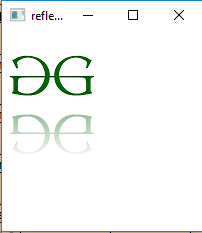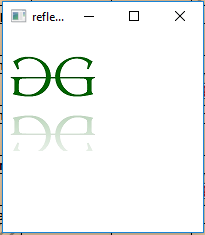# JavaFX | Reflection Class

Reflection class is a part of JavaFX. The Reflection class is used to add a reflected image below the actual image of the input value. The Reflected image will not respond to mouse events or the containment methods on the input.

Constructors of the class:

1. Reflection(): Creates a new Reflection Object.
2. Reflection(double topOffset, double fraction, double topOpacity, double bottomOpacity): Creates a new Reflection object with the specified topOffset, fraction, topOpacity and bottomOpacity.

Commonly Used Methods:

Method Explanation
getBottomOpacity() Returns the value of bottomOpacity
getTopOpacity() Returns the value of topOpacity
getFraction() Returns the fraction which the reflected image is of the real image
getTopOffset() Returns the value of top offset
getInput() Returns the value of property input
setBottomOpacity(double v) Sets the value of bottomOpacity
setTopOpacity(double v) Sets the value of topOpacity
setFraction(double v) Sets the fraction which the reflected image is of the real image
setTopOffset(double v) Sets the value of top offset
setInput(Effect v) Sets the value of property input

Below programs illustrate the use of Reflection class:

1. Java program to add a reflection to the image using the reflection class: In this program a FileInputStream is created and an image is taken as input from a file. An Image named image is created using the input from the file input stream. From the image, an image view object is created and it is added to the VBox. The VBox is then added to the scene and the scene is added to the stage. A Reflection effect is created and the effect is set to the image view using setEffect() function.

 `// Java program to add a reflection to  ` `// the image using the reflection class ` `import` `javafx.application.Application; ` `import` `javafx.scene.Scene; ` `import` `javafx.scene.control.*; ` `import` `javafx.scene.layout.*; ` `import` `javafx.stage.Stage; ` `import` `javafx.scene.image.*; ` `import` `javafx.scene.effect.*; ` `import` `java.io.*; ` `import` `javafx.event.ActionEvent; ` `import` `javafx.event.EventHandler; ` `import` `javafx.scene.Group; ` ` `  `public` `class` `reflection_1 ``extends` `Application { ` ` `  `    ``// launch the application ` `    ``public` `void` `start(Stage stage) ``throws` `Exception ` `    ``{ ` ` `  `        ``// set title for the stage ` `        ``stage.setTitle(``"reflection example"``); ` ` `  `        ``// create a input stream ` `        ``FileInputStream input = ``new` `FileInputStream(``"D:\\GFG.png"``); ` ` `  `        ``// create a image ` `        ``Image image = ``new` `Image(input); ` ` `  `        ``// create a image View ` `        ``ImageView imageview = ``new` `ImageView(image); ` ` `  `        ``// create a reflection effect ` `        ``Reflection reflection = ``new` `Reflection(); ` ` `  `        ``// set effect ` `        ``imageview.setEffect(reflection); ` ` `  `        ``// create a VBox ` `        ``VBox vbox = ``new` `VBox(imageview); ` ` `  `        ``// create a scene ` `        ``Scene scene = ``new` `Scene(vbox, ``200``, ``200``); ` ` `  `        ``// set the scene ` `        ``stage.setScene(scene); ` ` `  `        ``stage.show(); ` `    ``} ` ` `  `    ``// Main Method ` `    ``public` `static` `void` `main(String args[]) ` `    ``{ ` `        ``// launch the application ` `        ``launch(args); ` `    ``} ` `} `

Output:2. Java program to add a reflection to the image using the reflection class and set the top offset, top opacity, bottom opacity and fraction of image which will appear as a reflection: In this program a FileInputStream is created and an image is taken as input from a file. Image named image is created using the input from the file input stream. From the image, an image view object is created and it is added to the VBox . The VBox is then added to the scene and the scene is added to the stage. A Reflection effect is created and the effect is set to the image view using setEffect() function. The bottom Opacity, top Opacity, top offset, and fraction are set using the setBottomOpacity(), setTopOpacity(), setFraction(), and setTopOffset() function respectively.

 `// Java program to add a reflection to the image ` `// using the reflection class and set the top  ` `// offset, top opacity bottom opacity and fraction ` `// of image which will appear as reflection ` `import` `javafx.application.Application; ` `import` `javafx.scene.Scene; ` `import` `javafx.scene.control.*; ` `import` `javafx.scene.layout.*; ` `import` `javafx.stage.Stage; ` `import` `javafx.scene.image.*; ` `import` `javafx.scene.effect.*; ` `import` `java.io.*; ` `import` `javafx.event.ActionEvent; ` `import` `javafx.event.EventHandler; ` `import` `javafx.scene.Group; ` ` `  `public` `class` `reflection_2 ``extends` `Application { ` ` `  `    ``// launch the application ` `    ``public` `void` `start(Stage stage) ``throws` `Exception ` `    ``{ ` ` `  `        ``// set title for the stage ` `        ``stage.setTitle(``"reflection example"``); ` ` `  `        ``// create a input stream ` `        ``FileInputStream input = ``new` `FileInputStream(``"D:\\GFG.png"``); ` ` `  `        ``// create a image ` `        ``Image image = ``new` `Image(input); ` ` `  `        ``// create a image View ` `        ``ImageView imageview = ``new` `ImageView(image); ` ` `  `        ``// create a reflection effect ` `        ``Reflection reflection = ``new` `Reflection(); ` ` `  `        ``// set fraction ` `        ``reflection.setFraction(``0.6``); ` ` `  `        ``// set top Opacity ` `        ``reflection.setTopOpacity(``0.3``); ` ` `  `        ``// set bottom Opacity ` `        ``reflection.setBottomOpacity(``0.1``); ` ` `  `        ``// set top offset ` `        ``reflection.setTopOffset(``0.5``); ` ` `  `        ``// set effect ` `        ``imageview.setEffect(reflection); ` ` `  `        ``// create a VBox ` `        ``VBox vbox = ``new` `VBox(imageview); ` ` `  `        ``// create a scene ` `        ``Scene scene = ``new` `Scene(vbox, ``200``, ``200``); ` ` `  `        ``// set the scene ` `        ``stage.setScene(scene); ` ` `  `        ``stage.show(); ` `    ``} ` ` `  `    ``// Main Method ` `    ``public` `static` `void` `main(String args[]) ` `    ``{ ` ` `  `        ``// launch the application ` `        ``launch(args); ` `    ``} ` `} `

Output:Note: The above programs might not run in an online IDE. Please use an offline compiler.

My Personal Notes arrow_drop_upSecond year Department of Information Technology Jadavpur University

If you like GeeksforGeeks and would like to contribute, you can also write an article using contribute.geeksforgeeks.org or mail your article to contribute@geeksforgeeks.org. See your article appearing on the GeeksforGeeks main page and help other Geeks.

Please Improve this article if you find anything incorrect by clicking on the "Improve Article" button below.

Improved By : Akanksha_Rai

Article Tags :
Practice Tags :

Be the First to upvote.

Please write to us at contribute@geeksforgeeks.org to report any issue with the above content.